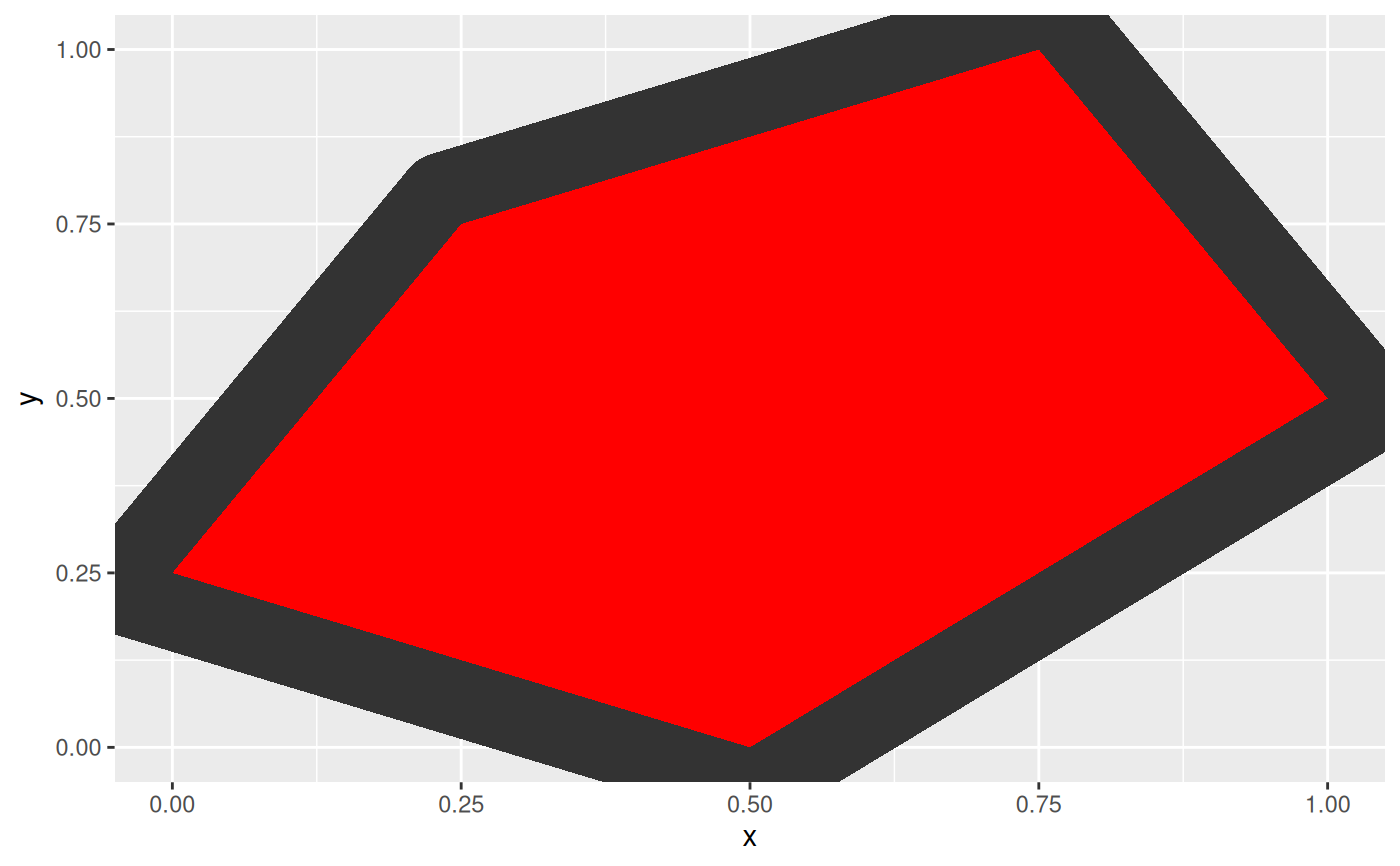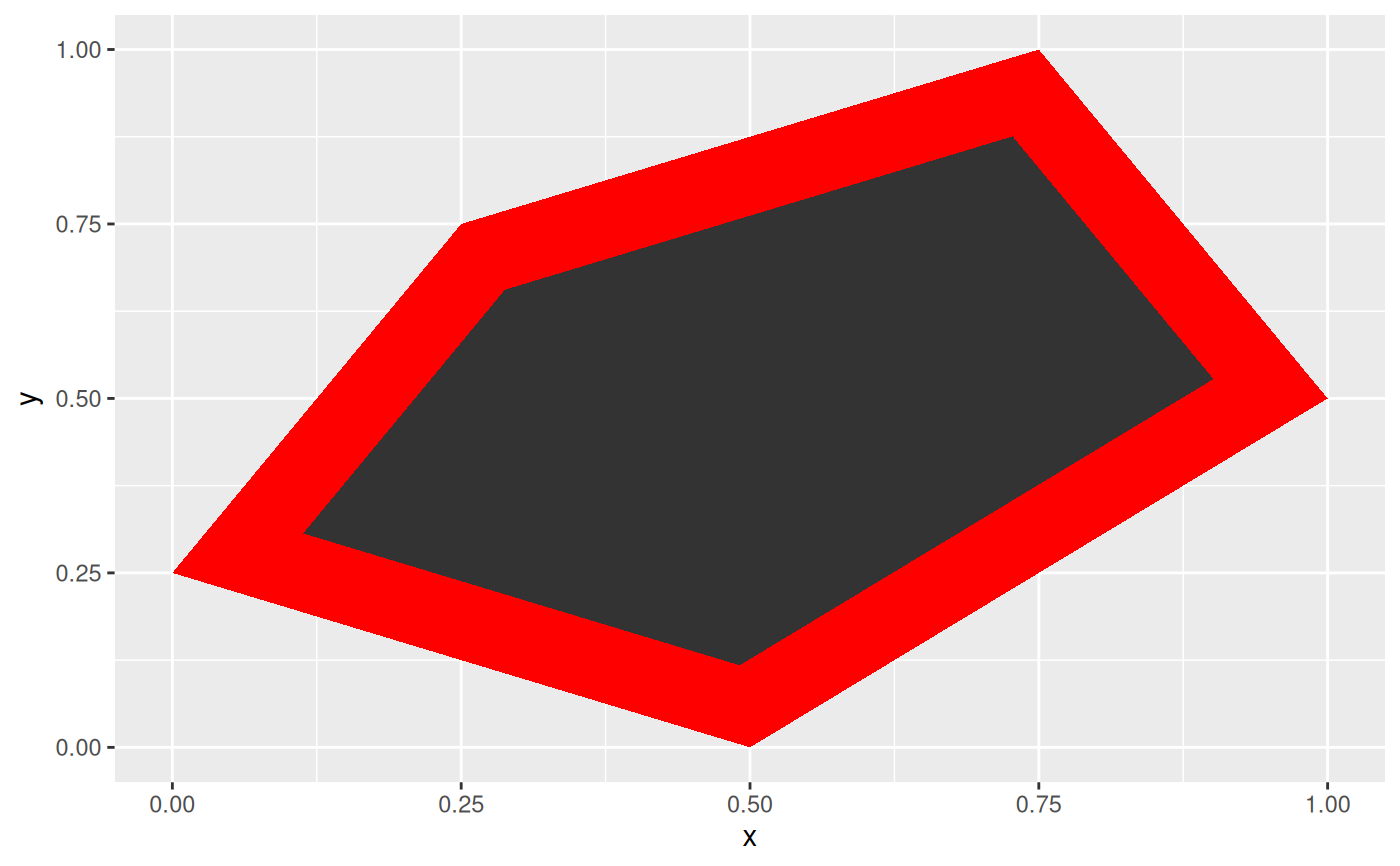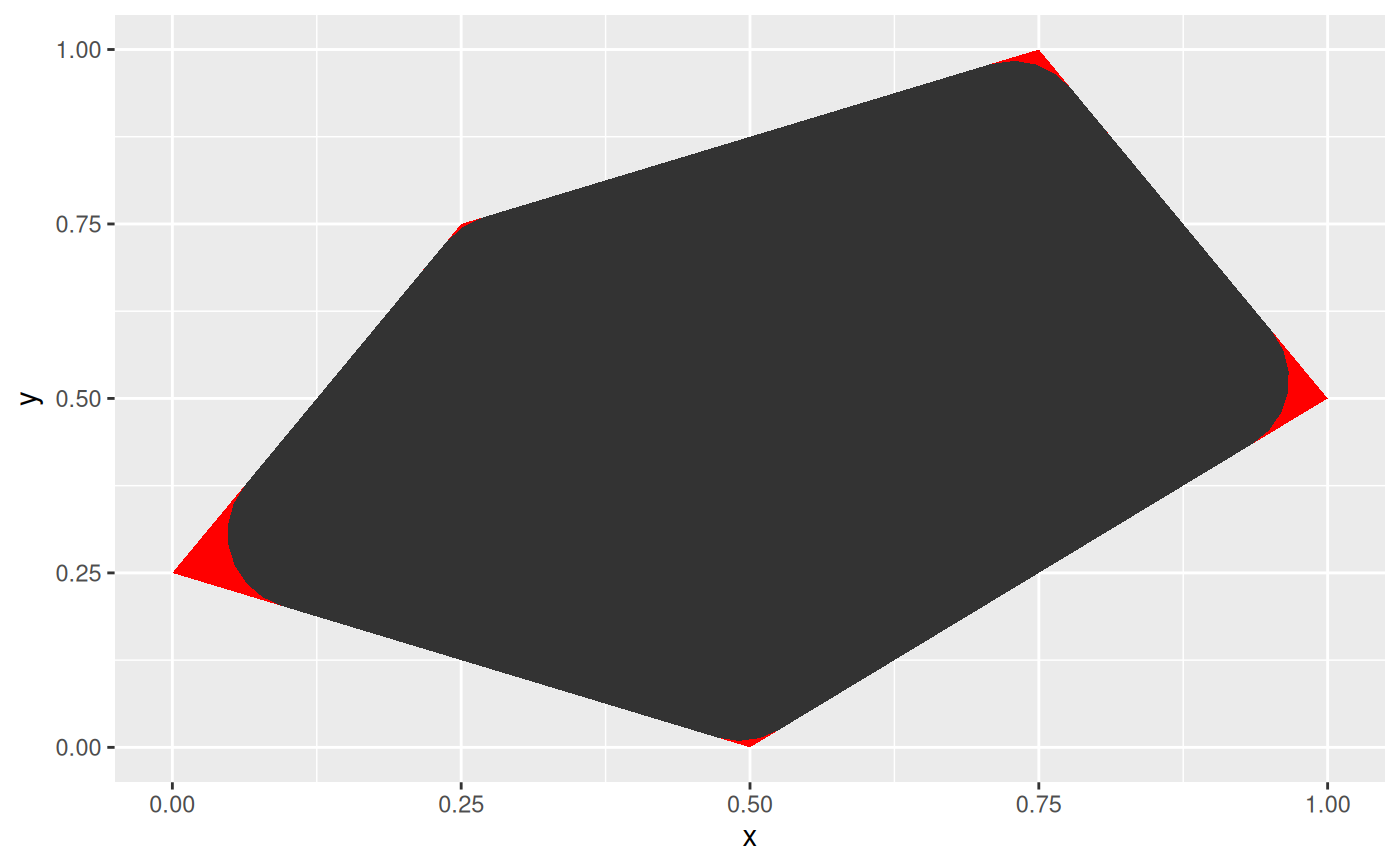This geom is a cousin of ggplot2::geom_polygon() with the added possibility of expanding or contracting the polygon by an absolute amount (e.g. 1 cm). Furthermore, it is possible to round the corners of the polygon, again by an absolute amount. The resulting geom reacts to resizing of the plot, so the expansion/contraction and corner radius will not get distorted. If no expansion/contraction or corner radius is specified, the geom falls back to geom_polygon so there is no performance penality in using this instead of geom_polygon.

geom_shape(
mapping = NULL,
data = NULL,
stat = "identity",
position = "identity",
expand = 0,
...,
na.rm = FALSE,
show.legend = NA,
inherit.aes = TRUE
)

## Arguments

mapping

Set of aesthetic mappings created by aes(). If specified and inherit.aes = TRUE (the default), it is combined with the default mapping at the top level of the plot. You must supply mapping if there is no plot mapping.

data

The data to be displayed in this layer. There are three options:

If NULL, the default, the data is inherited from the plot data as specified in the call to ggplot().

A data.frame, or other object, will override the plot data. All objects will be fortified to produce a data frame. See fortify() for which variables will be created.

A function will be called with a single argument, the plot data. The return value must be a data.frame, and will be used as the layer data. A function can be created from a formula (e.g. ~ head(.x, 10)).

stat

The statistical transformation to use on the data for this layer, either as a ggproto Geom subclass or as a string naming the stat stripped of the stat_ prefix (e.g. "count" rather than "stat_count")

position

Position adjustment, either as a string naming the adjustment (e.g. "jitter" to use position_jitter), or the result of a call to a position adjustment function. Use the latter if you need to change the settings of the adjustment.

expand

A numeric or unit vector of length one, specifying the expansion amount. Negative values will result in contraction instead. If the value is given as a numeric it will be understood as a proportion of the plot area width.

As expand but specifying the corner radius.

...

Other arguments passed on to layer(). These are often aesthetics, used to set an aesthetic to a fixed value, like colour = "red" or size = 3. They may also be parameters to the paired geom/stat.

na.rm

If FALSE, the default, missing values are removed with a warning. If TRUE, missing values are silently removed.

show.legend

logical. Should this layer be included in the legends? NA, the default, includes if any aesthetics are mapped. FALSE never includes, and TRUE always includes. It can also be a named logical vector to finely select the aesthetics to display.

inherit.aes

If FALSE, overrides the default aesthetics, rather than combining with them. This is most useful for helper functions that define both data and aesthetics and shouldn't inherit behaviour from the default plot specification, e.g. borders().

## Note

Some settings can result in the dissappearance of polygons, specifically when contracting or rounding corners with a relatively large amount. Also note that x and y scale limits does not take expansion into account and the resulting polygon might thus not fit into the plot.

## Aesthetics

geom_shape understand the following aesthetics (required aesthetics are in bold):

• x

• y

• color

• fill

• group

• size

• linetype

• alpha

## Author

Thomas Lin Pedersen

## Examples

shape <- data.frame(
x = c(0.5, 1, 0.75, 0.25, 0),
y = c(0, 0.5, 1, 0.75, 0.25)
)
# Expand and round
ggplot(shape, aes(x = x, y = y)) +
geom_shape(expand = unit(1, 'cm'), radius = unit(0.5, 'cm')) +
geom_polygon(fill = 'red')# Contract
ggplot(shape, aes(x = x, y = y)) +
geom_polygon(fill = 'red') +
geom_shape(expand = unit(-1, 'cm'))# Only round corners
ggplot(shape, aes(x = x, y = y)) +
geom_polygon(fill = 'red') +